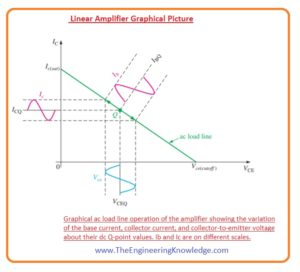Hello friends, I hope you all are doing great. In today’s tutorial, we will have a look at Describe amplifier operation. The biasing process of a transistor is completely dc operation. The main function of biasing is to discuss a Q point due to which change in current and voltage can exit according to the sinusoidal input waveform. In such an application in which less value voltage is amplified like radio transmission or antenna or microphone have small changing in Q point.

Amplifier created to control these less sinusoidal signals is usually known as a small-signal amplifier. In today’s post, we will have a detailed look at the amplifier operation. So let’s get started with Describe amplifier operation.

#### Describe Amplifier Operation

• As we know that for recognization of dc quantities non-italic uppercase subscripts are used like IC, IE, VC and VCE.
• But for lowercase italic subscripts identified the ac quantities such as root mean square, peal, and p-to-p current and voltage.
• For instance Ic, Ie, Ib, Vc and Vce.
• While lowercase quantities denoted by both lowercase letters and subscripts like ic, ie, ib and vce.
• The below figure defines the quantities for a certain voltage signal.• There are different values of current and voltage for the resistance circuit is analyze for ac point of view than for dc.
• Lowercase subscripts are used to define ac resistance parameters.
• For instance, Rc is denoted for ac collector resistor and RC is denoted for dc collector resistor.

#### Linear Amplifier

• Linear amplifier is such amplifier that amplifies the signal without any signal disturbance according to an input signal.
• The voltage divider biased transistor has a sinewave signal source is connected to the base through the capacitor C1 and a load connected with the collector through the capacitor C2. It is shown in the below figure.
• The coupled capacitor stops dc and that provides protection to the interior source resistance Rs, and the load resistance RL from varying the dc bias voltage at the bias voltage at collector and base terminals.
• The capacitor looks shorted with the signal voltage. The ac signal source changes the base voltage sinusoidally over its dc bias level, VBQ.
• The resultant change in base current generates a greater change in the collector current due to transistor current gain.
``````

Graphical Picture

• The above-discussed function can be described in the shape of a graph over the ac load line as shown in the below figure.
• The ac voltage at base generates a base current which changes over the Q-point on the ac load line as shown in the figure with the arrows.
• A line drawn from peak of base current to collector current axis and to VCE axis denoted the peak to peak changing of collector current a collector to emitter voltage as shown.
• The ac load line is different from the dc load line due to the effective acc collector resistor RL is connected in parallel
•  With the RC and is has value lesser than the dc collector resistor RC.